# LPV Approximation of Boost Converter Model

This example shows how to obtain a linear parameter varying (LPV) approximation of a Simscape™ Electrical™ model of a boost converter. The LPV representation allows quick analysis of average behavior at various operating conditions.

### Boost Converter Model

A Boost Converter circuit converts a DC voltage to another DC voltage by controlled chopping or switching of the source voltage. The request for a certain load voltage is translated into a corresponding requirement for the transistor duty cycle. The duty cycle modulation is typically several orders of magnitude slower than the switching frequency, which produces an average voltage with relatively small ripples, as shown in the following figure.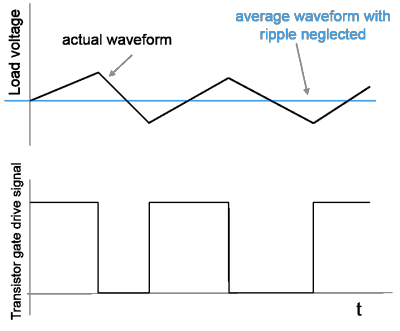In practice, there are also disturbances in the source voltageand the resistive loadaffecting the actual load voltage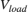.

```mdl = 'BoostConverterExampleModel'; open_system(mdl) ```The circuit in the model is characterized by high-frequency switching. The model uses a sample time of 25 ns. The Boost Converter block used in the model is a variant subsystem that implements two different versions of the converter dynamics. The model takes the duty cycle value as its only input and produces three outputs: inductor current, load current, and load voltage.

Due to the high-frequency switching and short sample time, the model simulates slowly.

### Batch Trimming and Linearization

In many applications, the average voltage delivered in response to a certain duty cycle profile is of interest. Such behavior is studied at time scales several decades larger than the fundamental sample time of the circuit. These average models for the circuit are derived by analytical considerations based on averaging of power dynamics over certain time periods. The `BoostConverterExampleModel` model implements such an average model of the circuit as its first variant, called AVG Voltage Model. This variant typically executes faster than the Low Level Model variant.

The average model is not a linear system. It shows nonlinear dependence on the duty cycle and the load variations. To produce faster simulation and to help with voltage stabilizing controller design, you can linearize the model at various duty cycle and load values.

For this example, use the snapshot-based trimming and linearization. The scheduling parameters are the duty cycle `d` and resistive load `R`. You trim and linearize the model for several values of the scheduling parameters.

For this example, select a span of 10-60% for the duty cycle variation and a span of 4-15 ohms for the load variation. Select five values in these ranges for each scheduling variable and linearization obtained at all possible combinations of their values.

```nD = 5; nR = 5; dspace = linspace(0.1,0.6,nD); % Values of d in 10%-60% range Rspace = linspace(4,15,nR); % Values of Rin 4-15 Ohms range [dgrid,Rgrid] = ndgrid(dspace,Rspace); % All combinations of d and R values ```

Create a parameter structure array for the scheduling parameters.

```params(1).Name = 'd'; params(1).Value = dgrid; params(2).Name = 'R'; params(2).Value = Rgrid; ```

Specify the number of model inputs, outputs, and states.

```ny = 3; nu = 1; nx = 2; ArraySize = size(dgrid); ```

A simulation of the model under various conditions shows that the model outputs settle down to their steady-state values before 0.01 s. Therefore, use t = 0.01s as the snapshot time.

Compute equilibrium operating points at the snapshot time using the `findop` function. This operation takes several minutes to finish.

```op = findop(mdl,0.01,params); ```

To linearize the model, first obtain the linearization input and output points from the model.

```io = getlinio(mdl); ```

Configure the linearization options to store linearization offsets.

```opt = linearizeOptions('StoreOffsets', true); ```

Linearize the model at the operating points in array `op`.

```[linsys,~,info] = linearize(mdl,op,io,params,opt); ```

Extract offsets from the linearization results.

```offsets = getOffsetsForLPV(info); yoff = offsets.y; xoff = offsets.x; uoff = offsets.u; ```

Plot the linear system array.

```bodemag(linsys) grid on ```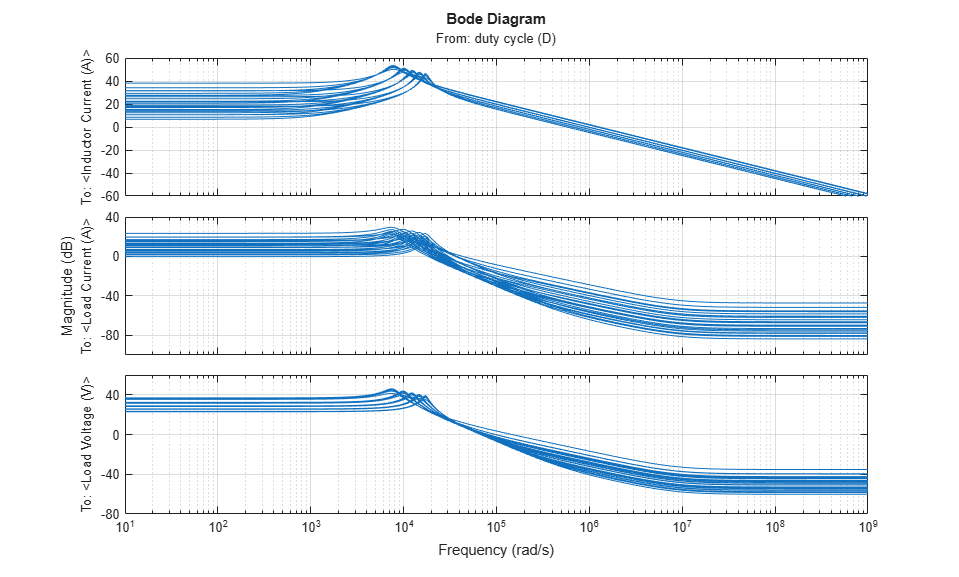### LPV Simulation

`linsys` is an array of 25 linear state-space models, each with 1 input, 3 outputs, and 2 states. The models are discrete-time with a sample time of 25 ns. The bode plot shows significant variation in dynamics over the grid of scheduling parameters.

You can configure an LPV System block using the linear system array and the accompanying offset data (`uoff`, `yoff`, and `xoff`). The resulting LPV model serves as a linear system array approximation of the average dynamics. The `BoostConverterLPVModel` model uses such an LPV approximation.

```lpvmdl = 'BoostConverterLPVModel'; open_system(lpvmdl) ```For simulating the model, use an input profile for the duty cycle that roughly covers its scheduling range. Also, vary the resistive load to simulate load disturbances.

Generate the duty cycle profile `din`.

```t = linspace(0,.05,1e3)'; din = 0.25*sin(2*pi*t*100)+0.25; din(500:end) = din(500:end)+.1; ```

Generate the resistive load profile `rin`.

```rin = linspace(4,12,length(t))'; rin(500:end) = rin(500:end)+3; rin(100:200) = 6.6; ```

Plot the scheduling parameter profiles.

```yyaxis left plot(t,din) xlabel('Time (s)') ylabel('Duty Cycle') yyaxis right plot(t,rin) ylabel('Resistive Load (Ohm)') title('Scheduling Parameter Profiles for Simulation') ```The code for generating the above signals has been added to the model `PreLoadFcn` callback for independent loading and execution. To override these settings and try your own, overwrite this data in the MATLAB® workspace.

Simulate the LPV model and view the resulting output.

```sim(lpvmdl,'StopTime','0.004'); open_system('BoostConverterLPVModel/Outputs') ```The LPV model simulates significantly faster than the original `BoostConverterExampleModel` model.

To compare these simulation results with the original boost converter model simulation, use the `BoostConverterResponseComparison` model. This model uses the Boost Converter block configured to use the high-fidelity Low Level Model variant. It also contains the LPV System block. You can view the responses for both systems in the model scopes.

```linsysd = c2d(linsys,Ts*1e4); mdl = 'BoostConverterResponseComparison'; open_system(mdl) ```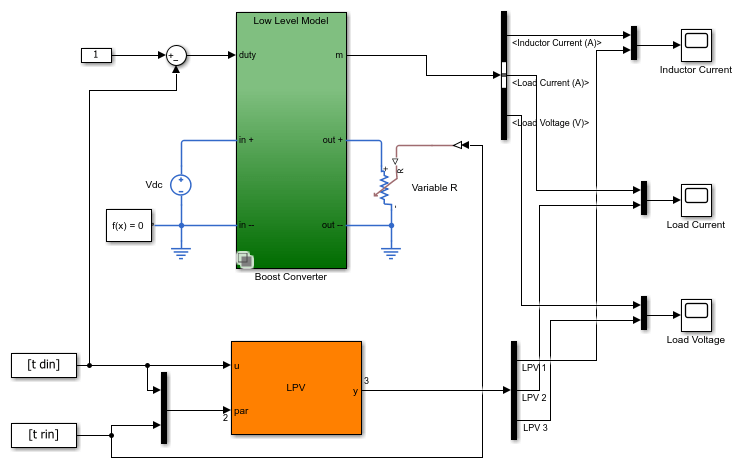Simulate the model. The simulation runs quite slowly due to the fast switching dynamics in high-fidelity model. Uncomment the following code to simulate the model.

```% sim(mdl); ```

The following figures show example simulation results for the inductor current, load current, and load voltage.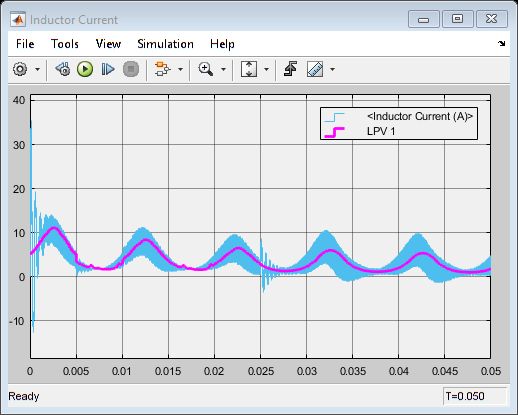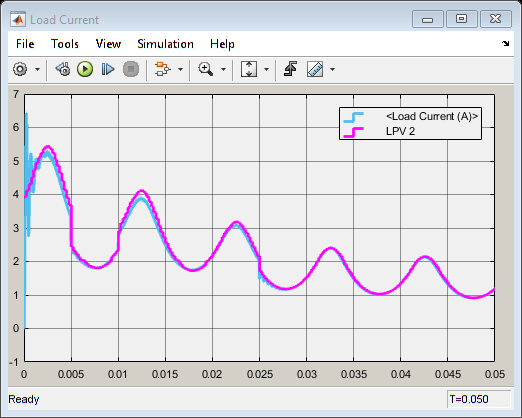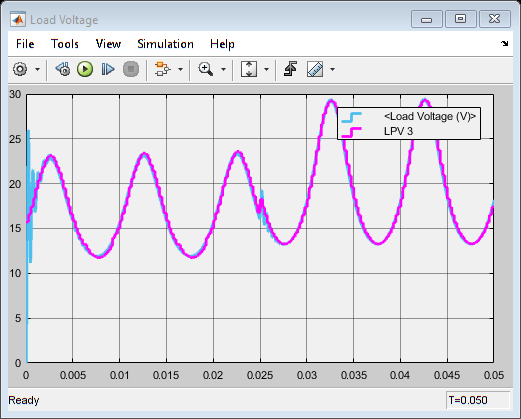While the LPV model consumes less memory and simulates significantly faster than the high-fidelity model, it is able to emulate the average behavior of the boost converter.